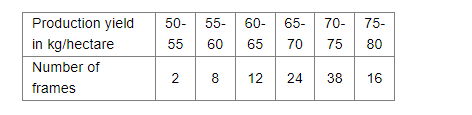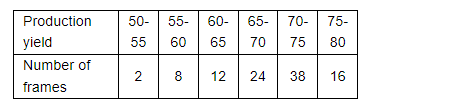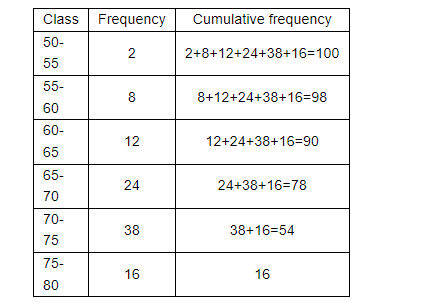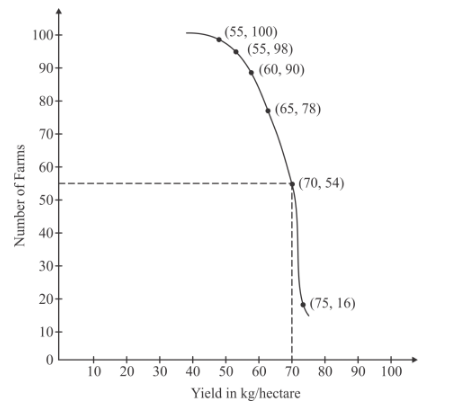# The following table gives the production yield`
Question:

The following table gives the production yield per hectare of wheat of 100 farms of a village.Change the above distribution to more than type distribution and draw its ogive.

Solution:

We have the following distributionWe have to change the above distribution in to the more than type distribution and we have to draw its ogive.

We have the following procedure to find the more than type distributionTo draw its ogive, take the number of frames on y-axis and production yield on x-axis. Mark the points (50,100), (55, 98), (60-90), (65, 78), (70, 54), and (75, 16) on the
xy-plane. Now these points are joined by free hand.